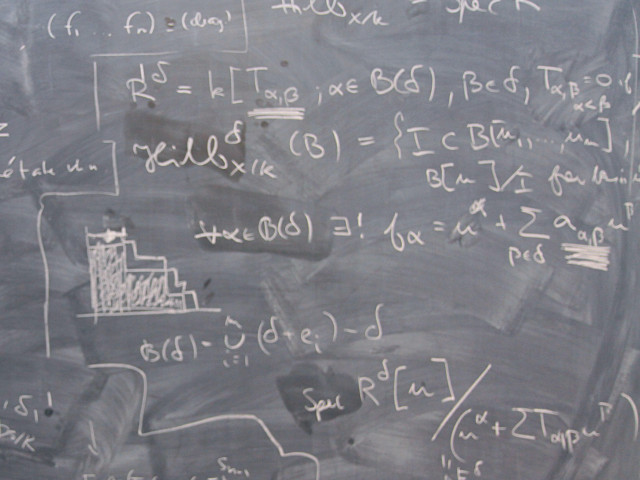# DN2266 Mathematical Models, Analysis and Simulation Part 1 7.5 credits

#### Matematiska modeller, analys och simulering del 1A second course in numerical methods with a mathematical emphasis offering applied and numerical mathematics useful for scientific and engineering modeling. Emphasis on close connection between the properties of mathematical models and their successful numerical treatment.

### Offering and execution

Course offering missing for current semester as well as for previous and coming semesters

## Course information

### Content and learning outcomes

#### Course contents *

Linear algebra, equilibrium and minimization problems. Applications on networks. Duality and calculus of variation, essential and natural boundary conditions. Systems of ordinary differential equations, linear and nonlinear. Phase plane, stability, bifurcation. Numerical methods for the solution of nonlinear systems and differential equations. Applications on mechanical and ecological systems.

Solution of systems of linear equations. Symmetrical positive definite matrices. Minimization problems. Eigenvalues and dynamical systems.

Assignments: One assignment every second week, from paper and pencil work to parameter studies of dynamical models in ecology and mechanics.

#### Intended learning outcomes *

The overall goal of the course is to give basic knowledge of applied and numerical mathematics useful for scientific and engineering modeling. Especially, the close connection between the properties of mathematical models and their successful numerical treatment is emphasized.

This understanding means that after the course you should be able to

• identify and describe discrete equilibrium models using a network approach;
• relate equilibrium problems to minimum principles and solve simple constraint minimization problems via the Lagrange multiplier approach;
• formulate variational problems starting from simple physical principles and derive the corresponding Euler-Lagrange equation such that you can derive basic equations for continuous equilibrium problems in one, two, and three space dimensions;
• model (spatially discrete) time-dependent systems by ordinary differential equations;
• investigate the stability of autonomous systems and explore geometrically the phase space of 2D dynamical systems using analytical and numerical tools;
• derive asymptotic expansions for certain simple singular perturbation problems;
• understand the relation between convergence, consistency, and stability of numerical methods;
• understand essential properties of, and proof error estimations for, numerical methods for solving stationary and instationary problems such that you can compare different methods and select suitable algorithms for given problems;
• analyze and select iterative methods for large linear systems.

#### Course Disposition

No information inserted

### Literature and preparations

#### Specific prerequisites *

Single course students: 90 university credits including 45 university credits in Mathematics or Information Technology. English B, or equivalent.

#### Recommended prerequisites

DN1212 or DN1240 or corresponding and experiences in programming in Matlab.

#### Equipment

No information inserted

#### Literature

Meddelas senast 4 veckor före kursstart på kursens hemsida. Tidigare läsår användes G. Strang: Computational Science and Engineering, Wellesley-Cambridge.

### Examination and completion

A, B, C, D, E, FX, F

#### Examination *

• TEN1 - Examination, 3.8 credits, Grading scale: A, B, C, D, E, FX, F

Based on recommendation from KTH’s coordinator for disabilities, the examiner will decide how to adapt an examination for students with documented disability.

The examiner may apply another examination format when re-examining individual students.

In this course all the regulations of the code of honor at the School of Computer science and Communication apply, see: http://www.kth.se/csc/student/hederskodex/1.17237?l=en_UK.

#### Other requirements for final grade *

Examination (TEN1; 3,8 university credits).
Assignments (LAB1; 3,7 university credits).

#### Opportunity to complete the requirements via supplementary examination

No information inserted

#### Opportunity to raise an approved grade via renewed examination

No information inserted

### Further information

#### Course web

Further information about the course can be found on the Course web at the link below. Information on the Course web will later be moved to this site.

Course web DN2266

SCI/Mathematics

Mathematics

Second cycle